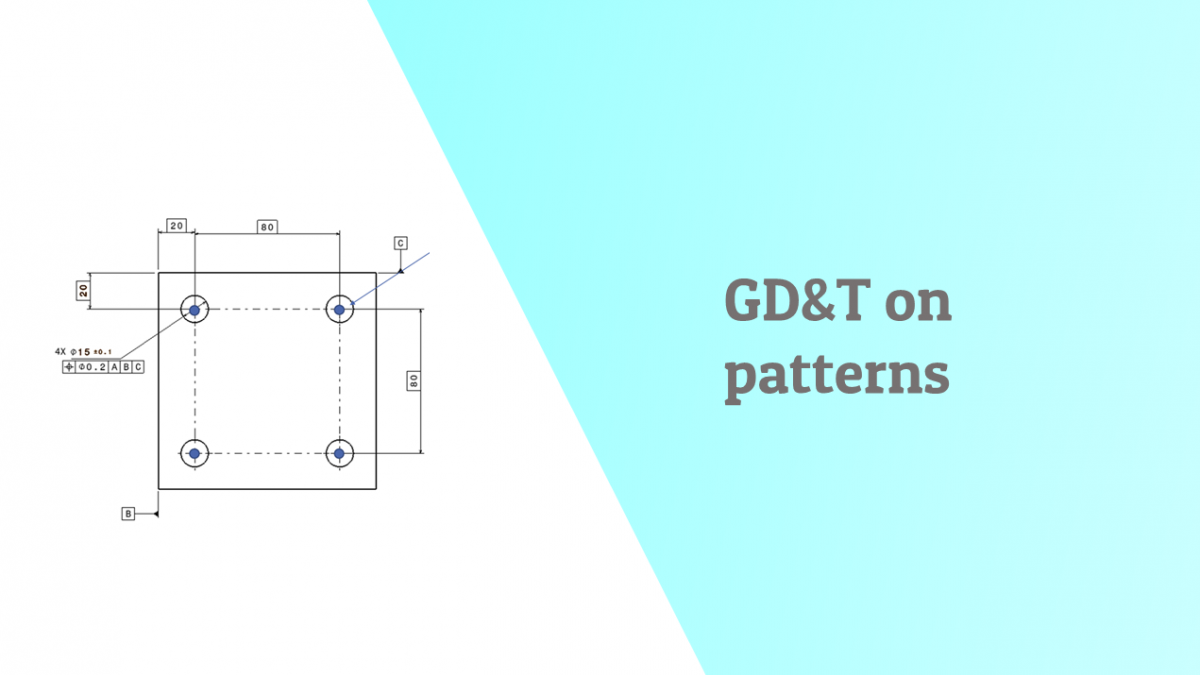# GD&T applied to patterns of featuresHow to interpret the meaning of a GD&T callout on a pattern of features? What are the tolerance zones like?

When there are multiple features in a pattern then the meaning of the GD&T scheme changes

Here is an example:

• The feature control frame says that there 7 features which are in a pattern and have to be located with respect to datum A, B C as per the basic dimensions and with respect to the basic distance in between them
• Controls perpendicularity orientation of each hole with datum A
• Locates the pattern within the geometric tolerance zone with respect to datum B and C
• Points of the pattern can only vary within the blue zone.
• The tolerance zone is called as Pattern locating tolerance zone.
• If there was no “4X’ then the position control would only apply to a single hole which is the first and not others.

Simplifies the GD&T drawing avoids repetitive annotations

Example no 2 shows a rectangular pattern of holes

A manufactured part with exaggerated form is shown here . the four plus signs indicate the centres of holes and the datum reference frame is show as the co ordinate system at top right edge of part .

Analytical definition of tolerance zones :

Each tolerance zone is a circle and those circles are locating a distance with respect to the origin which is the origin of datum reference frame.

Hence by finding the equations for each circle we can arrive the condition to satisfy each tolerance zone requirement.

Assumption:

• Only verifying position of hole in 2D
• Considering negligible variation in perpendicularity of axis

These are the equations of each tolerance zone with the condition for acceptance.

If we have a measured manufactured actual part and replace x and y values then the equations should be satisfied for acceptance.

Categories: Tolerancing